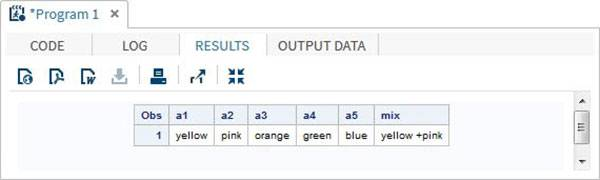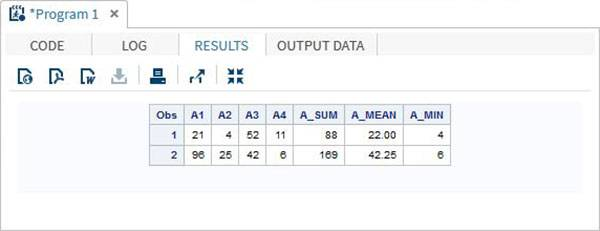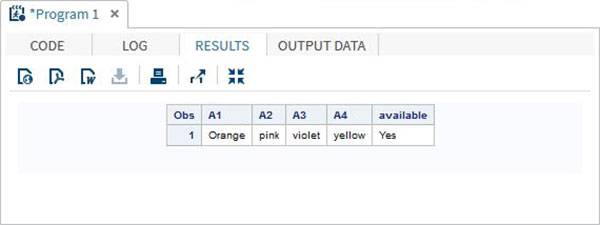# SAS数组

SAS中的数组用于使用索引值存储和检索一系列值。 索引表示保留存储区中的位置。

## 句法

```ARRAY ARRAY-NAME(SUBSCRIPT) (\$) VARIABLE-LIST ARRAY-VALUES
```

• ARRAY是声明数组的SAS关键字。
• ARRAY-NAME是遵循与变量名称相同的规则的数组的名称。
• SUBSCRIPT是数组要存储的值的数量。
• （\$）是一个可选参数，仅当数组要存储字符值时使用。
• VARIABLE-LIST是变量的可选列表，它们是数组值的占位符。
• ARRAY-VALUES是存储在数组中的实际值。 它们可以在这里声明，或者可以从文件或dataline中读取。

### 数组声明示例

```# Declare an array of length 5 named AGE with values.
ARRAY AGE (12 18 5 62 44);

# Declare an array of length 5 named COUNTRIES with values starting at index 0.
ARRAY COUNTRIES(0:8) A B C D E F G H I;

# Declare an array of length 5 named QUESTS which contain character values.
ARRAY QUESTS(1:5) \$ Q1-Q5;

# Declare an array of required length as per the number of values supplied.
ARRAY ANSWER(*) A1-A100;
```

## 访问数组值

```DATA array_example;
INPUT a1 \$ a2 \$ a3 \$ a4 \$ a5 \$;
ARRAY colours(5) \$ a1-a5;
mix = a1||'+'||a2;
DATALINES;
yello pink orange green blue
;
RUN;
PROC PRINT DATA=array_example;
RUN;
```### 使用OF运算符

```DATA array_example_OF;
INPUT A1 A2 A3 A4;
ARRAY A(4) A1-A4;
A_SUM=SUM(OF A(*));
A_MEAN=MEAN(OF A(*));
A_MIN=MIN(OF A(*));
DATALINES;
21 4 52 11
96 25 42 6
;
RUN;
PROC PRINT DATA=array_example_OF;
RUN;
```### 使用IN运算符

```DATA array_in_example;
INPUT A1 \$ A2 \$ A3 \$ A4 \$;
ARRAY COLOURS(4) A1-A4;
IF 'yellow' IN COLOURS THEN available='Yes';ELSE available='No';
DATALINES;
Orange pink violet yellow
;
RUN;
PROC PRINT DATA=array_in_example;
RUN;
```## 您可能还喜欢：